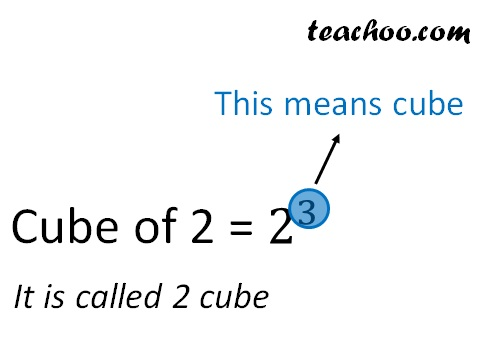Cubes

Chapter 6 Class 8 Cubes and Cube Roots
Concept wise

Number = 2

Cube of number = 2 3

= 2 × 2 × 2

= 8

Cube of a number is a number which we get when a number is multiplied by itself 3 times.

Here, 8 is a cube number

They are sometimes also called perfect cubesLet’s do some examples

 Number Cube 1 1 × 1 × 1 = 1 2 2 × 2 × 2 = 8 3 3 × 3 × 3 = 27 4 4 × 4 × 4 = 64 5 5 × 5 × 5 = 125 6 6 × 6 × 6 = 216 7 7 × 7 × 7 = 343 8 8 × 8 × 8 = 512 9 9 × 9 × 9 = 729 10 10 × 10 × 10 = 1000

Thus, 1, 8, 27, 64, 125, 216, 343, 512, 729, 1000 are cube numbers

## Is 1 a cube number?

Cube numbers is a number which we get when a number is multiplied by itself 3 times.

So, 1, 8, 27, 64, …. …. are cube numbers

Hence, 1 is a cube number

Learn in your speed, with individual attention - Teachoo Maths 1-on-1 Class